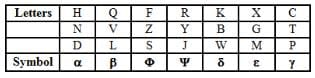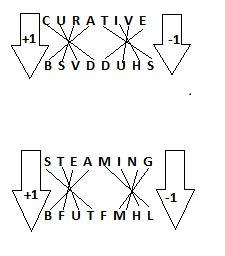# Test: Coding - Decoding- 2

## 20 Questions MCQ Test IBPS PO Prelims- Study Material, Online Tests, Previous Year | Test: Coding - Decoding- 2

Description
Attempt Test: Coding - Decoding- 2 | 20 questions in 15 minutes | Mock test for Banking Exams preparation | Free important questions MCQ to study IBPS PO Prelims- Study Material, Online Tests, Previous Year for Banking Exams Exam | Download free PDF with solutions
QUESTION: 1

### If REUNION LIMITED is written as QAOMEIM KELESAC, how will SCHOOL be written?

Solution:

C is the correct option.In the given coded word each constant is substituted by preceding constant and each vowel is also substituted by preceding vowel. Hence, SCHOOL will be codes as RBGIIK.

QUESTION: 2

### If ROME BOMBAY is written as SUNI CUNCEZ, how will ASIA will be written?

Solution:

Vowel is coded by the next vowel and consonant is coded by the next consonant. Therefore, using the same pattern 'ASIA will' be coded as ETOE.

QUESTION: 3

### If TEMPORARY is written as YRAROPMET, how will PERMANENT will be written?

Solution:

All the alphabets are reversed in TEMPORARY. Hence, PERMANENT would be TNENAMREP.

QUESTION: 4

If TEMPORARY is written as GVNKLIZIB how will PERMANENT be written?

Solution:

The formula is = 27 - no. of the alphabet on which it is placed. = the answer

27 - 20(T) = 7(G)

Similarly the whole word will be done like this

Also

27 - (P)16 = 11 (K)

27 - (E)5  = 22(V)

And so on...

QUESTION: 5

In a certain code language, 123 means ‘I prefer coffee’, 356 means ‘They dislike coffee’, and 589 means ‘They want tea’, which digit stands for ‘dislike’?

Solution:

In the first and second statements, the common code digit is '3' and the common word is 'coffee'.
So, '3' means 'coffee'.
In the second and third statements, the common code digit '5' and the common word is 'they'.
So, '5' means 'they'.
Thus, in the second statements, '6' means 'dislike'.

QUESTION: 6

If in a certain language BLEMISH is coded as AODPHVG, how is CHAPTER coded in that code?

Solution:

Here, the coded letters at odd places are one place before and those at even places are three places ahead of the corresponding letters in the given word.
Similarly, CHAPTER is coded as BKZSSHQ.

QUESTION: 7

In a certain code “ Nee Chi Tee” means “Ring the bell”, “Ki chi Ba” means “Bell is black” and “Su nee ki la” means “The pen is red. Which code stands for ‘Ring’?

Solution:

In 'nee chi tee' and 'ki chi ba' the common word is chi and in their meaning the common word is bell. Hence, chi means bell.
Similarly in 'nee chi tee' and 'su nee ki la' nee is common and in their respective meanings 'the' is common, which means nee=the. The remaining word in nee chi tee is tee, which means the remaing word ring.

QUESTION: 8

If man is called lady, lady is called girl. Girl is called boy, boy is called butler and butler is called rogue, who will serve in a restaurant.

Solution:

A 'butler' serves in a restaurant but 'butler' is called 'rogue'.
So a 'rouge' will serve in the restaurant.

QUESTION: 9

If in a certain language 943 is coded as BED and 12448 is coded as SWEET. How is 492311 coded in that languages?

Solution:

The numbers are coded as shown:
9431 2 8

BEDSWT

i.e. 4 as E,
9 as B,
2 as W,
3 as D and
1 as S.
So, 492311 is coded as EBWDSS.

QUESTION: 10

If TEMPORARY is written as BEDGFIAIG, how will PERMANENT be written?

Solution:

By comparing TEMPORARY with BEDGFIAIG, the coded Letters for each letter will be:
P - G
E - E
R - I
M - D
A - A
N - ?
E - E
N - ?
T - B
Since, none of the options mentioned suits with the coded letters, the option 'D' will be correct.

QUESTION: 11

In a certain code language BLUNDER is written as LBNURED. Then how will QUICKER be written in that code?

Solution:

The consecutive alphabets places are interchanged and the last 3 alphabets and reversed
i.e in QUICKER
Q and U are interchanged and form UQ
I and C are interchanged and form CI
KER, the last alphabets will be reversed to form REK.
Combining all, we get UQCIREK.

QUESTION: 12

In a certain code language

I. ‘Do No Pa’ means ‘Amit is lucky’.

II.  ‘Po Cho To’ means ‘She was there’.

III. Po No Bo’ means ‘She is beautiful’.

Then which of the following is the code for ‘lucky’?

Solution:

In the sentence, 'Do No Pa' and 'Po No Bo', the word No is common. Moreover, in their explanations 'is' common as well. Hence, here 'No' means 'is'. Since, the meaning of 'Do' and 'Pa' is ambiguous, the word 'lucky' could mean either of the two.

QUESTION: 13

How will you write ‘is’ in a certain code?

1. Old is Gold means ‘cha ta ni’
2. Gold to Sold means ‘si ni ki’
3. me fold old means ‘ti pa ta’
Solution:

Here,

By comparing 1 and 2, we find

Gold = ni
By comparing 1 and 3, we find

old = ta
Hence, 'is' should be 'cha'.

QUESTION: 14

In a certain code language the word FUNCTION is written as UCINHPVQ. How will the word REGULATE be written in that language?

Solution:

In the code, FUNCTION is written as UCHINHPVQ.

Here, the alphabets at even places have been written as it is and the alphabets at odd places have been moved 2 places ahead.

Hence, REGULATE is EUAETINV

QUESTION: 15

The consonants of English alphabet have been coded by using Greek alphabets as given in the table and vowels by using different digits.If any vowel is neither in the beginning nor at the end, it is coded as ‘3’. If any vowel is at the beginning or at the end, it is coded as ‘7’. However, if the same vowel is placed at both beginning and the end, it is coded as ‘9’ respectively.

Q. How will the following letter groups be coded?

ERLMTNO

Solution:

A vowel is at the beginning or at the end, it is coded as ‘7’.

7 _ _ _ _ _ 7

=> From the table, we can substitute letters from symbols

R --> Ψ

L --> β

M --> ε

T --> γ

N --> α

ERLMTNO = 7Ψβεγα7

QUESTION: 16

In a certain language if A is written as 2, B as 4 and C as 6 what do the figure 12, 10, 10, 8 stands for?

Solution:

12   10   10   8
F      E     E    D

QUESTION: 17

The consonants of English alphabet have been coded by using Greek alphabets as given in the table and vowels by using different digits.If any vowel is neither in the beginning nor at the end, it is coded as ‘3’. If any vowel is at the beginning or at the end, it is coded as ‘7’. However, if the same vowel is placed at both beginning and the end, it is coded as ‘9’ respectively.

Q. How will the following letter groups be coded?

IYQHZEXI

Solution:

As instructed in the question, the same vowels placed at both beginning and end should be coded as 9.

Hence, the code should be:

9ψβαφ9ε9

None of the options match the instruction, hence the answer is option d.

QUESTION: 18

The consonants of English alphabet have been coded by using Greek alphabets as given in the table and vowels by using different digits.If any vowel is neither in the beginning nor at the end, it is coded as ‘3’. If any vowel is at the beginning or at the end, it is coded as ‘7’. However, if the same vowel is placed at both beginning and the end, it is coded as ‘9’ respectively.

Q. How will the following letter groups be coded?

AVILFKPU

Solution:

As per the instructions, AVILFKPU should be coded as:

7β7βφδγ7

QUESTION: 19

The consonants of English alphabet have been coded by using Greek alphabets as given in the table and vowels by using different digits.If any vowel is neither in the beginning nor at the end, it is coded as ‘3’. If any vowel is at the beginning or at the end, it is coded as ‘7’. However, if the same vowel is placed at both beginning and the end, it is coded as ‘9’ respectively.

Q. How will the following letter groups be coded?

UGHIBOCSU

Solution:

As instructed in the question, the same vowels placed at both beginning and end should be coded as 9.

Hence, the code should be:

9εα9δ9γφ9

None of the options match the instruction, hence the answer is option d.

QUESTION: 20

How will the following letter groups be coded?

In a certain code CURATIVE is written as BSVDDUHS. How is STEAMING written in that code?

Solution:

The correct option is A.Use Code STAYHOME200 and get INR 200 additional OFF Use Coupon Code